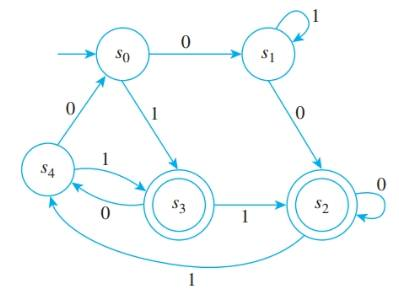Chapter 12.2, Problem 11ES### Discrete Mathematics With Applicat...

5th Edition
EPP + 1 other
ISBN: 9781337694193

#### Solutions

Chapter
Section### Discrete Mathematics With Applicat...

5th Edition
EPP + 1 other
ISBN: 9781337694193
Textbook Problem
1 views

# A finite-state automaton A given by the transition diagram on the next page, has next-stale function N and eventual-state function N*.a. Find N ( s 3 ,   0 ) and N ( s 2 ,   1 ) . b. Find N ( s 0 ,   0 ) and N ( s 4 ,   1 ) . c. Find N * ( s 0 ,   010011 ) and N * ( s 3 ,   01101 ) .d. Find N * ( s 0 ,   1111 ) and N * ( s 2 ,   00111 ) .

To determine

(a)

To find:

N(s3,0) and N(s2,1).

Explanation

Given information:

A finite-state automation A, given by a transition diagram has next state function N and eventual-state function N*.

Calculation:

Since, from the diagram s3 connecting to s4 with input 0.

Hence, N(s3,0)=s4

And, s2 connecting to s4 with input 1

To determine

(b)

To find:

N(s0,0) and N(s4,1).

To determine

(c)

To find:

N*(s0,010011) and N*(s3,01101).

To determine

(d)

To find:

N*(s0,1111) and N*(s2,00111).

### Still sussing out bartleby?

Check out a sample textbook solution.

See a sample solution

#### The Solution to Your Study Problems

Bartleby provides explanations to thousands of textbook problems written by our experts, many with advanced degrees!

Get Started